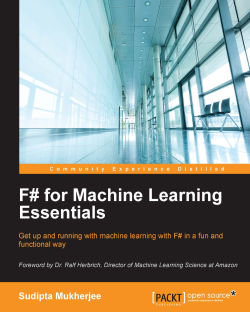# F# for Machine Learning Essentials4 (1 reviews total)
By Sudipta Mukherjee
What do you get with a Packt Subscription?

• Constantly updated with 100+ new titles each month
• Breadth and depth in over 1,000+ technologies

The F# functional programming language enables developers to write simple code to solve complex problems. With F#, developers create consistent and predictable programs that are easier to test and reuse, simpler to parallelize, and are less prone to bugs.

If you want to learn how to use F# to build machine learning systems, then this is the book you want.

Starting with an introduction to the several categories on machine learning, you will quickly learn to implement time-tested, supervised learning algorithms. You will gradually move on to solving problems on predicting housing pricing using Regression Analysis. You will then learn to use Accord.NET to implement SVM techniques and clustering. You will also learn to build a recommender system for your e-commerce site from scratch. Finally, you will dive into advanced topics such as implementing neural network algorithms while performing sentiment analysis on your data.

Publication date:
February 2016
Publisher
Packt
Pages
194
ISBN
9781783989348

## Chapter 1. Introduction to Machine Learning

"To learn is to discover patterns."

You have been using products that employ machine learning, but maybe you've never realized that the systems or programs that you have been using, use machine learning under the hood. Most of what machine learning does today is inspired by sci-fi movies. Machine learning scientists and researchers are on a perpetual quest to make the gap between the sci-fi movies and the reality disappear. Learning about machine learning algorithms can be fun.

This is going to be a very practical book about machine learning. Throughout the book I will be using several machine learning frameworks adopted by the industry. So I will cut the theory of machine learning short and will get away with just enough to implement it. My objective in this chapter is to get you excited about machine learning by showing how you can use these techniques to solve real world problems.

## Objective

After reading this chapter, you will be able to understand the different terminologies used in machine learning and the process of performing machine learning activities. Also, you will be able to look at a problem statement and immediately identify which problem domain the problem belongs to; whether it is a classification or a regression problem, and such. You will find connections between seemingly disparate sets of problems. You will also find basic intuition behind some of the major algorithms used in machine learning today. Finally, I wrap up this chapter with a motivating example of identifying hand written digits using a supervised learning algorithm. This is analogous to your `Hello world` program.

### Getting in touch

I have created the following Twitter account for you (my dear reader) to get in touch with me. If you want to ask a question, post errata, or just have a suggestion, tag this twitter ID and I will surely get back as soon as I can.

I will post contents here that will augment the content in the book.

### Different areas where machine learning is being used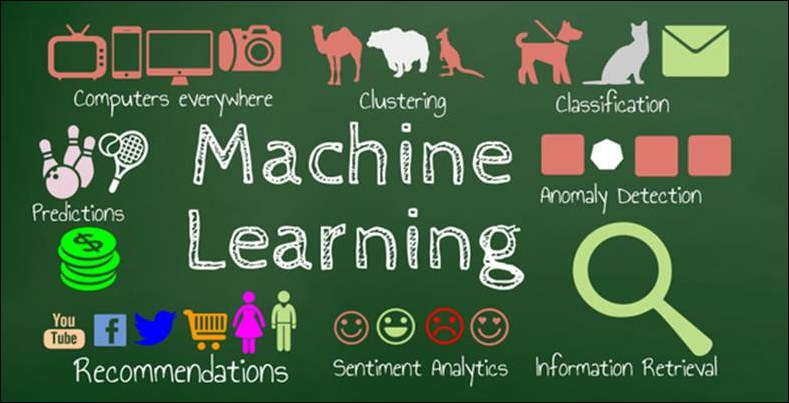The preceding image shows some of the areas where machine learning techniques are used extensively. In this book, you will learn about most of these usages.

Machines learn almost the same way as we humans do. We learn in three different ways.

As kids our parents taught us the alphabets and thus we can distinguish between the A's and H's. The same is true with machines. Machines are also taught the same way to recognize characters. This is known as supervised learning.

While growing up, we taught ourselves the differences between the teddy bear toy and an actual bear. This is known as unsupervised learning, because there is no supervision required in the process of the learning. The main type of unsupervised learning is called clustering; that's the art of finding groups in unlabeled datasets. Clustering has several applications, one of them being customer base segmentation.

Remember those days when you first learnt how to take the stairs? You probably fell many times before successfully taking the stairs. However, each time you fell, you learnt something useful that helped you later. So your learning got re-enforced every time you fell. This process is known as reinforcement learning. Ever saw those funky robots crawling uneven terrains like humans. That's the result of re-enforcement learning. This is a very active topic of research.

Whenever you shop online at Amazon or on other sites, the site recommends back to you other stuff that you might be interested in. This is done by a set of algorithms known as recommender systems.

Machine learning is very heavily used to determine whether suspicious credit card transactions are fraudulent or not. The technique used is popularly known as anomaly detection. Anomaly detection works on the assumption that most of the entries are proper and that the entry that is far (also called an outlier) from the other entries is probably fraudulent.

In the coming decade, machine learning is going to be very commonplace and it's about time to democratize the machine learning techniques. In the next few sections, I will give you a few examples where these different types of machine learning algorithms are used to solve several problems.

## Why use F#?

F# is an open source, functional-first, general purpose programming language and is particularly suitable for developing mathematical models that are an integral part of machine learning algorithm development.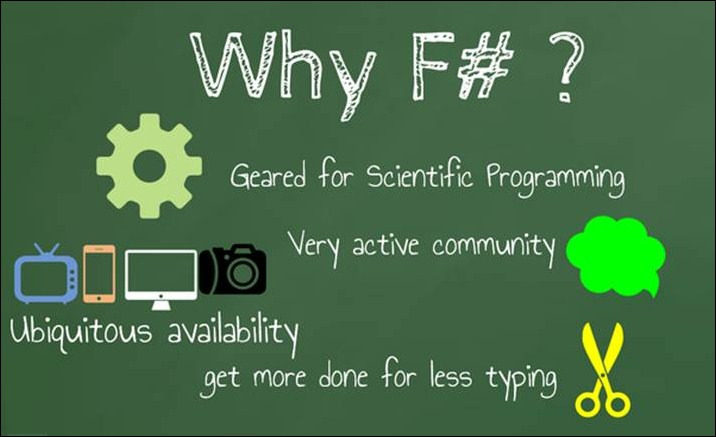Code written in F# is generally very expressive and is close to its actual algorithm description. That's why you shall see more and more mathematically inclined domains adopting F#.

At every stage of a machine learning activity, F# has a feature or an API to help. Following are the major steps in a machine learning activity:

Major step in machine learning activity

How F# can help

Data Acquisition

F# type providers are great at it. (Refer to http://blogs.msdn.com/b/dsyme/archive/2013/01/30/twelve-type-providers-in-pictures.aspx)

F# can help you get the data from the following resources using F# type providers:

• Databases (SQL Server and such)

• XML

• CSV

• JSON

• World Bank

• Cloud Storages

• Hive

Data Scrubbing/Data Cleansing

F# list comprehensions are perfect for this task.

Deedle (http://bluemountaincapital.github.io/Deedle/) is an API written in F#, primarily for exploratory data analysis. This framework also has lot of features that can help in the data cleansing phase.

Learning the Model

WekaSharp is an F# wrapper on top of Weka to help with machine learning tasks such as regression, clustering, and so on.

Accord.NET is a massive framework for performing a very diverse set of machine learning.

Data Visualization

F# charts are very interactive and intuitive to easily generate high quality charts. Also, there are several APIs, such as FsPlot, that take the pain of conforming to standards when it comes to plugging data to visualization.

F# has a way to name a variable the way you want if you wrap it with double back quotes like—"my variable". This feature can make the code much more readable.

### Supervised machine learning

Supervised machine learning algorithms are mostly broadly classified into two major categories: classification and regression.

Supervised machine learning algorithms work with labeled datasets. This means that the algorithm takes a lot of labeled data sets, where the data represents the instance and the label represents the class of the data. Sometimes these labels are finite in number and sometimes they are continuous numbers. When the labels belong to a finite set, then the problem of identifying the label of an unknown/new instance is known as a classification problem. On the other hand, if the label is a continuous number, then the problem of finding the continuous value for a new instance is known as a regression problem. Given a set of records for cancer patients, with test results and labels (B for benign and M for malignant) predicting whether a new patient's case is B or M, is a classification problem. On the other hand, predicting the price of a house, given the area in square feet and the number of bedrooms in the house, is an example of a regression problem.

I found the following analogy to geometry very useful when thinking about these algorithms.

Let's say you have two points in 2D. You can calculate the Euclidean distance between those two and if that distance is small, you can conclude that those points are close to each other. In other words, if those two points represent two cities in a country, you might conclude that they are in the same district.

Now if you extrapolate this theory to the N dimension, you can immediately see that any measurement can be represented as a point with the N dimension or as a vector of size N and a label can be associated with it. Then an algorithm can be deployed to learn the associativity or the pattern, and thus it learns to predict the label for an unseen/unknown/new instance represented in the similar format.

#### Training and test dataset/corpus

The phase when an algorithm runs over a labeled data set is known as training, and the labeled data is known as training dataset. Sometimes it is loosely referred to as training corpus. Later in the process, the algorithm must be tested with similar un-labeled datasets or for which the label is hidden from the algorithm. This dataset is known as test dataset or test corpus. Typically, an 80-20 split is used to generate the training and test set from a randomly shuffled labeled data set. This means that 80% of the randomly shuffled labeled data is generally treated as training data and the remaining 20% as test data.

#### Some motivating real life examples of supervised learning

Supervised learning algorithms have several applications. Following are some of those. This is by no means a comprehensive list, but it is indicative.

• Classification

• Spam filtering in your mailbox

• Cancer prediction from the previous patient records

• Identifying objects in images/videos

• Identifying flowers from measurements

• Identifying hand written digits on cheques

• Predicting whether there will be a traffic jam in a city

• Making recommendations to the users based on their and similar user's preferences

• Regression

• Predicting the price of houses based on several features, such as the number of bedrooms in the house

• Finding cause-effect relationships between several variables

• Supervised learning algorithms

• Nearest Neighbor algorithm

• Support Vector Machine

• Decision Tree

• Linear Regression

• Logistic Regression

• Naïve Bayes Classifier

• Neural Networks

• Recommender systems algorithms

• Anomaly Detection

• Sentiment Analysis

In the next few sections, I will walk you through the overview of a few of these algorithms and their mathematical basis. However, we will get away with as minimal math as possible, since the objective of the book is to help you use machine learning in the real settings.

#### Nearest Neighbour algorithm (a.k.a k-NN algorithm)

As the name suggests, k-Nearest Neighbor is an algorithm that works on the distance between two projected points. It relies on the distance of k-nearest neighbors (thus the name) to determine the class/category of the unknown/new test data.

As the name suggests the nearest neighbor algorithm relies on the distance of two data points projected in N-Dimensional space. Let's take a popular example where the k-NN can be used to determine the class. The dataset https://archive.ics.uci.edu/ml/machine-learning-databases/breast-cancer-wisconsin/wdbc.data stores data about several patients who were either unfortunate and diagnosed as "Malignant" cases (which are represented as M in the dataset), or were fortunate and diagnosed as "Benign" (non-harmful/non-cancerous) cases (which are represented as B in the dataset). If you want to understand what all the other fields mean, take a look at https://archive.ics.uci.edu/ml/machine-learning-databases/breast-cancer-wisconsin/wdbc.names.

Now the question is, given a new entry with all the other records except the tag M or B, can we predict that? In ML terminology, this value "M" or "B" is sometimes referred to as "class tag" or just "class". The task of a classification algorithm is to determine this class for a new data point. K-NN does this in the following way: it measures the distance from the given data to all the training data and then takes into consideration the classes for only the k-nearest neighbors to determine the class of the new entry. So for the current case, if more than 50% of the k-nearest neighbors is of class "B", then k-NN will conclude that the new entry is of type "B".

#### Distance metrics

The distance metric used is generally Euclidean, that you learnt in high school. For example, given two points in 3D.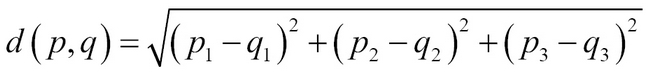In this preceding example,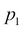and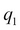denote their values in the X axis,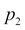and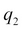denote their values in the Y axis, and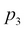and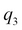denote their values in the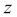axis.

Extrapolating this, we get the following formula for calculating the distance in N dimension: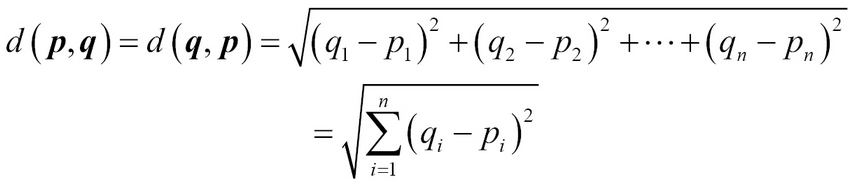Thus, after calculating the distance from all the training set data, we can create a list of tuples with the distance and the class, as follows. This list is made for the sake of demonstration. This is not calculated from the actual data.

Distance from test/new data

Class/Tag/Category

0.34235

B

0.45343

B

1.34233

B

6.23433

M

66.3435

M

Let's assume that k is set to be 4. Now for each k, we take into consideration the class. So for the first three entries, we found that the class is B and for the last one, it is M. Since the number of B's is more than the number of M's, k-NN will conclude that the new patient's data is of type B.

#### Decision tree algorithms

Decision tree is a set of classification algorithm that uses this approach to determine the class of an unknown entry. As the name suggests, a decision tree is a tree where the nodes depict the questions asked and the edges represent the decisions (yes/no). Leaf nodes determine the final class of the unknown entry. Following is a classic textbook example of a decision tree: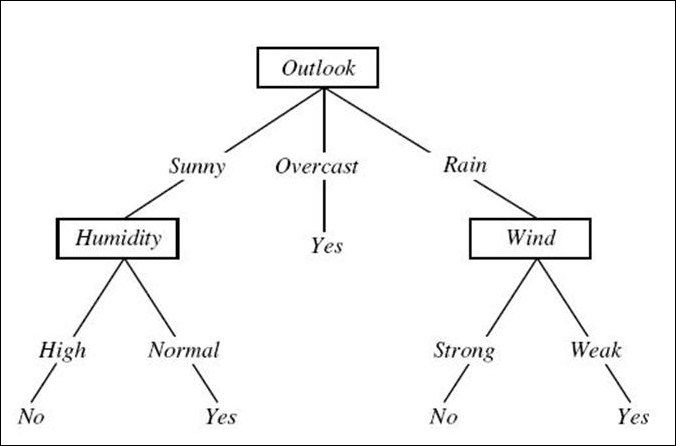The preceding figure depicts the decision whether we can play lawn tennis or not, based on several attributes such as Outlook, Humidity, and Wind. Now the question that you may have is why outlook was chosen as the root node of the tree. The reason was that by choosing outlook as the first feature/attribute to split the dataset, the outcomes were split more evenly than if the split had been done with other attributes such as "humidity" or "wind".

The process of finding the attribute that can split the dataset more evenly than others is guided by entropy. Lesser the entropy, better the parameter. Entropy is known as the measure of information gain. It is calculated by the following formula: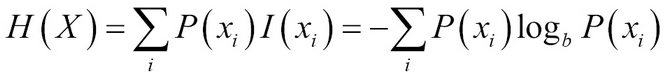Here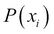stands for the probability of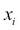and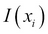denotes the information gain.

Let's take the example of tennis dataset from Weka. Following is the file in the CSV format:

```outlook,temperature,humidity,wind,playTennis
sunny, hot, high, weak, no
sunny, hot, high, strong, no
overcast, hot, high, weak, yes
rain, mild, high, weak, yes
rain,cool, normal, weak, yes
rain, cool, normal, strong, no
overcast, cool, normal, strong, yes
sunny, mild, high, weak, no
sunny, cool, normal, weak, yes
rain, mild, normal, weak, yes
sunny, mild, normal, strong, yes
overcast, mild, high, strong, yes
overcast, hot, normal, weak, yes
rain, mild, high, strong, no```

You can see from the dataset that out of 14 instances (there are 14 rows in the file), 5 instances had the value `no` for `playTennis` and 9 instances had the value `yes`. Thus, the overall information is given by the following formula: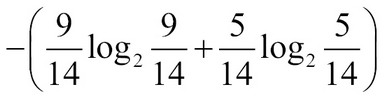This roughly evaluates to 0.94. Now from the next steps, we must pick the attribute that maximizes the information gain. Information gain is denoted as the difference between the total entropy and the entropy calculated for each possible split.

Let's go with one example. For the outlook attribute, there are three possible values: `rain`, `sunny`, and `overcast`, and for each of these values, the value of the attribute `playTennis` is either `no` or `yes`.

For `rain`, out of `5` instances, `3` instances have the value `yes` for the attribute `playTennis`; thus, the entropy is as follows: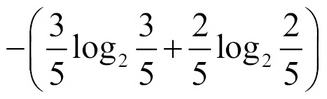This is equal to 0.97.

For `overcast`, every instance has the value `yes`: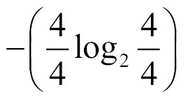This is equal to 0.0.

For `sunny`, out of `5` instances, only `2` have the value `yes`: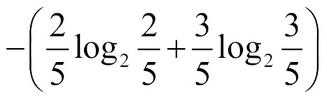So the expected new entropy is given by the following formula: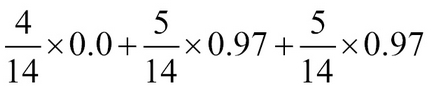This is roughly equal to 0.69. If you follow these steps for the other attributes, you will find that the new entropies are like as follows:

Attribute

Entropy

Information gain

outlook

0.69

0.94 – 0.69 => 0.25

temperature

0.91

0.94 – 0.91 => 0.03

humidity

0.724

0.94 – 0.725 => 0.215

windy

0.87

0.94 – 0.87 => 0.07

So the highest information gain is attained if we split the dataset based on the outlook attribute.

Sometimes multiple trees are constructed by generating a random subset of all the available features. This technique is known as random forest.

##### Linear regression

Regression is used to predict the target value of the real valued variable. For example, let's say we have data about the number of bedrooms and the total area of many houses in a locality. We also have their prices listed as follows:

Number of Bedrooms

Total Area in square feet

Price

2

1150

2300000

3

2500

5600000

3

1780

4571030

4

3000

9000000

Now let's say we have this data in a real estate site's database and we want to create a feature to predict the price of a new house with three bedrooms and total area of 1650 square feet.

Linear regression is used to solve these types of problems. As you can see, these types of problems are pretty common.

In linear regression, you start with a model where you represent the target variable—the variable for which you want to predict the value. A polynomial model is selected that minimizes the least square error (this will be explained later in the chapter). Let me walk you through this example.

Each row of the available data can be represented as a tuple where the first few elements represent the value of the known/input parameters and the last parameter shows the value of the price (the target variable). So taking inspiration from mathematics, we can represent the unknown with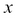and known as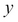. Thus, each row can be represented as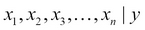where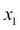to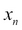represent the parameters (the total area and the number of bedrooms) andrepresents the target value (the price of the house). Linear regression works on a model where y is represented with the x values.

The hypothesis is represented by an equation as the following. Hereand theta denotes the input parameters (the number of bedrooms and the total area in square feet) and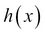represents the predicted value of the new house.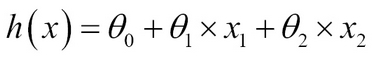Note that this hypothesis is still a polynomial model and we are just using two features: the number of bedrooms and the total area represented by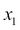and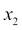.

So the square error is calculated by the following formula: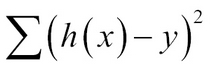The task of linear regression is to choose a set of values for the coefficients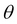which minimizes this error. The algorithm that minimizes this error is called gradient descent or batch gradient descent. You will learn more about it in Chapter 2, Linear Regression.

##### Logistic regression

Unlike linear regression, logistic regression predicts a Boolean value indicating the class/tag/category of the target variable. Logistic regression is one of the most popular binary classifiers and is modelled by the equation that follows.and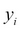stands for the independent input variables and their classes/tags respectively. Logistic regression is discussed at length in Chapter 3, Classification Techniques.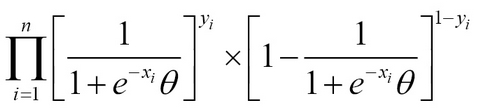##### Recommender systems

Whenever you buy something from the web (say Amazon), it recommends you stuff that you might find interesting and might eventually buy as well. This is the result of recommender system. Let's take the following example of a movie rating:

Movie

Bob

Lucy

Jane

Jennifer

Jacob

Paper Towns

1

3

4

2

1

Focus

2

5

?

3

2

Cinderella

2

?

4

2

3

Jurrasic World

3

1

4

5

?

Die Hard

5

?

4

5

5

So in this toy example, we have 5 users and they have rated 5 movies. But not all the users have rated all the movies. For example, Jane hasn't rated "Focus" and Jacob hasn't rated "Jurassic World". The task of a recommender system is to initially guess what would be the ratings for the movies that aren't rated by the user and then recommend movies that have a guessed rating which is beyond a threshold (say 3).

There are several algorithms to solve this problem. One popular algorithm is known as collaborative filtering where the algorithm takes clues from the other user ratings. You will learn more about this in Chapter 5, Collaborative Filtering.

## Unsupervised learning

As the name suggests, unlike supervised learning, unsupervised learning works on data that is not labeled or that doesn't have a category associated with each training example.

Unsupervised learning is used to understand data segmentation based on a few features of the data. For example, a supermarket might want to understand how many different types of customers they have. For that, they can use the following two features:

• The number of visits per month (number of times the customer shows up)

• The average bill amount

The initial data that the supermarket had might look like the following in a spreadsheet: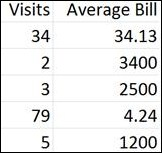So the data plotted in these 2 dimensions, after being clustered, might look like this following image: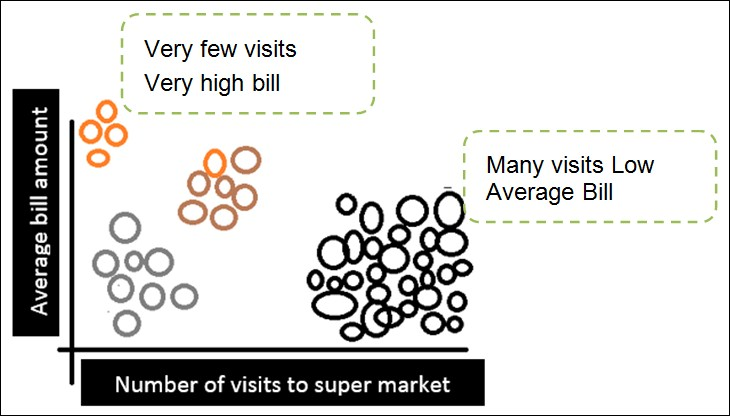Here you see that there are 4 types of people with two extreme cases that have been annotated in the preceding image. Those who are very thorough and disciplinarian and know what they want, go to the store very few times and buy what they want, and generally their bills are very high. The vast majority falls under the basket where people make many trips (kind of like darting into a super market for a packet of chips, maybe) but their bills are really low. This type of information is crucial for the super market because they can optimize their operations based on these data.

This type of segmenting task has a special name in machine learning. It is called "clustering". There are several clustering algorithms and K Means Clustering is quite popular. The only flip side of k Means Clustering is that the number of possible clusters has to be told in the beginning.

## Machine learning frameworks

I will be using the following machine learning frameworks to solve some of the real problems throughout the book:

You are much better off using these frameworks than creating your own because a lot of work has been done and they are used in the industry. So if you pick up using these frameworks along the way while learning about machine learning algorithms in general, that's a great thing. You will be in luck.

## Machine learning for fun and profit

Machine learning requires a lot of data and most of the time you, as the developer of an algorithm, will not have the time to synthesize or obtain good data. However, you are in luck. Kaggle does that for you. Kaggle is a website where companies host several machine learning problems and they provide training and test data to test your algorithm. Some competitions are linked with employment. So if your model stands out, you stand a chance to interview with the company that hosted the competition. Here is a short list of companies that are using kaggle for their data science/machine learning problems: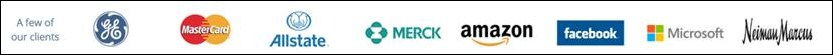The next section gets you started with a kaggle competition; getting the data and solving it.

## Recognizing handwritten digits – your "Hello World" ML program

Handwritten digits can be recognized with k-nearest neighbor algorithm.

Each handwritten digit is written on a 28*28 matrix. So there are 28*28 -> 784 pixels and each of these are represented as a single column of the dataset. Thus, the dataset has 785 columns. The first column is the label/digit and the remaining 784 values are the pixel values.

Following is a small example. Let's say, if we're to imagine this example as an 8 by 8 matrix, we would have something like the following figure for the digit 2: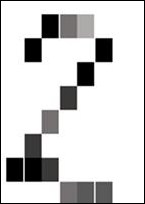A matrix can be represented as a 2-D array where each pixel is represented by each cell. However, any 2-D array can be visually unwrapped to be a 1-D array where the length of the array is the product of the length and the breadth of the array. For example, for the 8 by 8 matrix, the size of the single dimensional array will be 64. Now if we store several images and their 2D matrix representations, we will have something as shown in the following spreadsheet: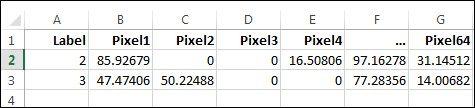The header Label denotes the number and the remaining values are the pixel values. Lesser the pixel values, the darker the cell is in the pictorial representation of the number 2, as shown previously.

In this program, you will write code to solve the digit recognizer challenge from Kaggle, available at:

Once you get there, download the data and save it in some folder. We will be using the `train.csv` file (You can get the file from www.kaggle.com/c/digit-recognizer/data) for training our classifier. In this example, you will implement the k nearest neighbor algorithm from scratch, and then deploy this algorithm to recognize the digit.

For your convenience, I have pasted the code at https://gist.github.com/sudipto80/72e6e56d07110baf4d4d.

Following are the steps to create the classifier:

1. Open Visual Studio 2013.

2. Create a new project: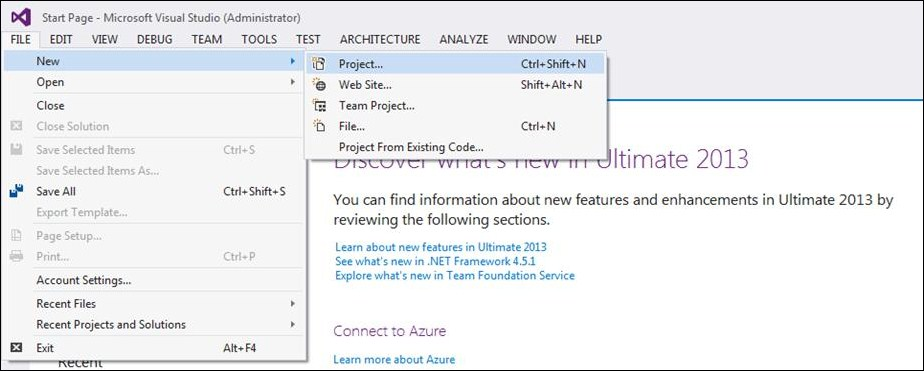3. Select F# and give a name for the console app: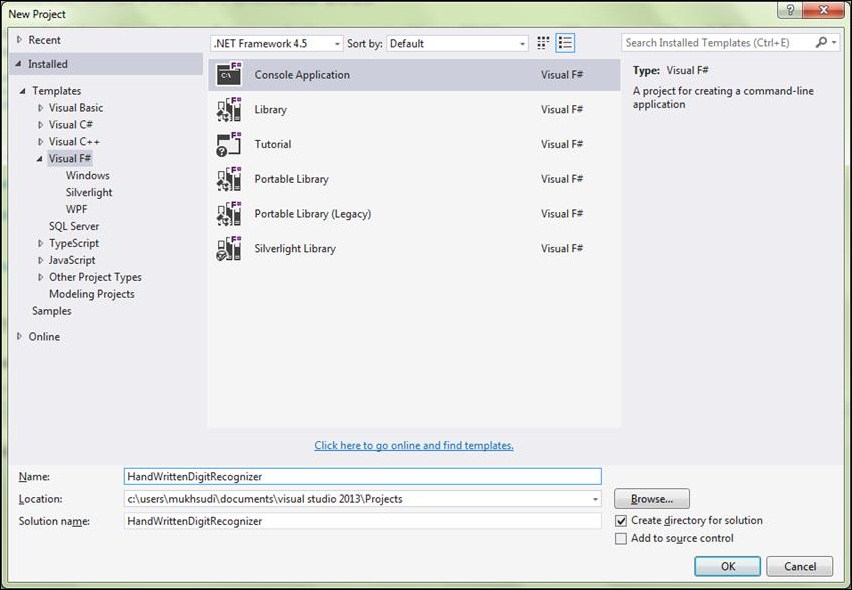4. Once you create the project by clicking "OK", your `program.fs` file will look as the following image: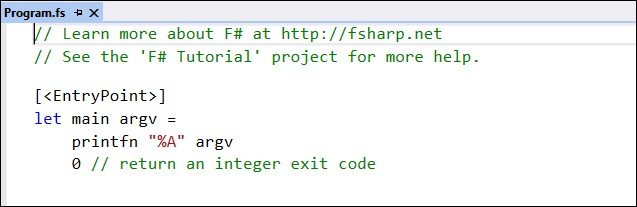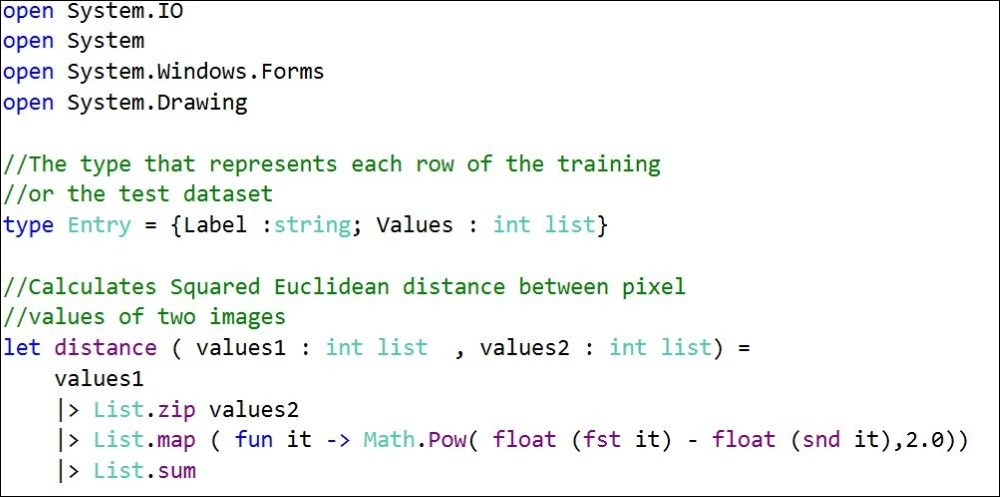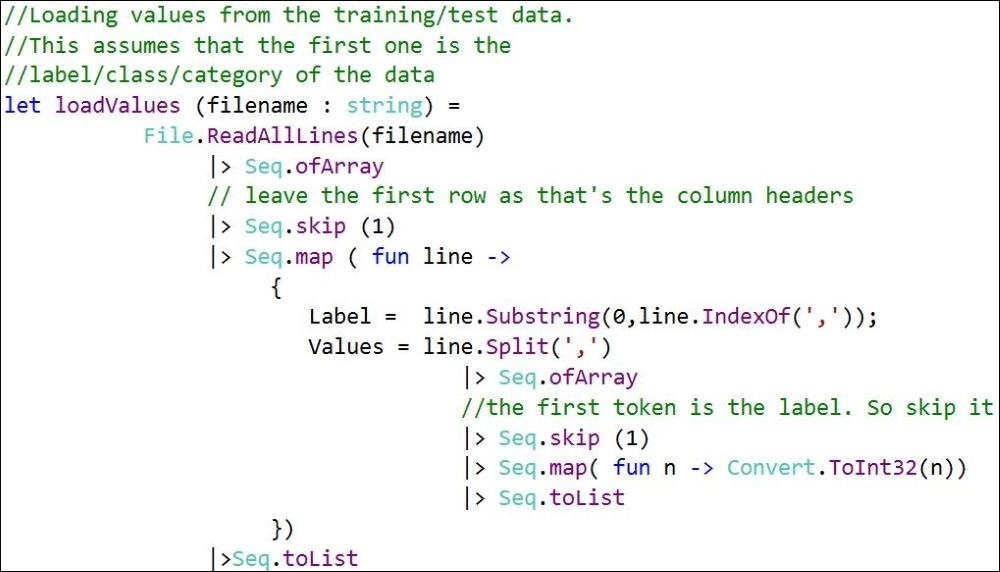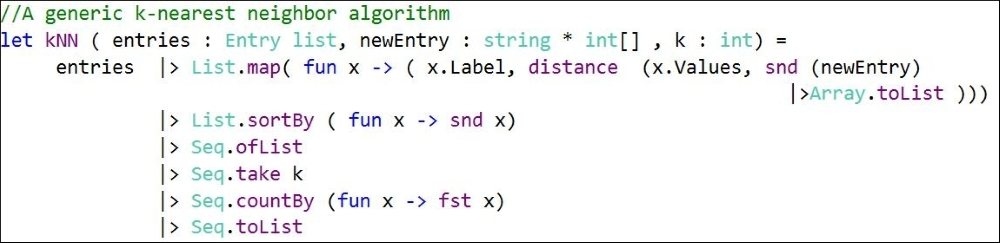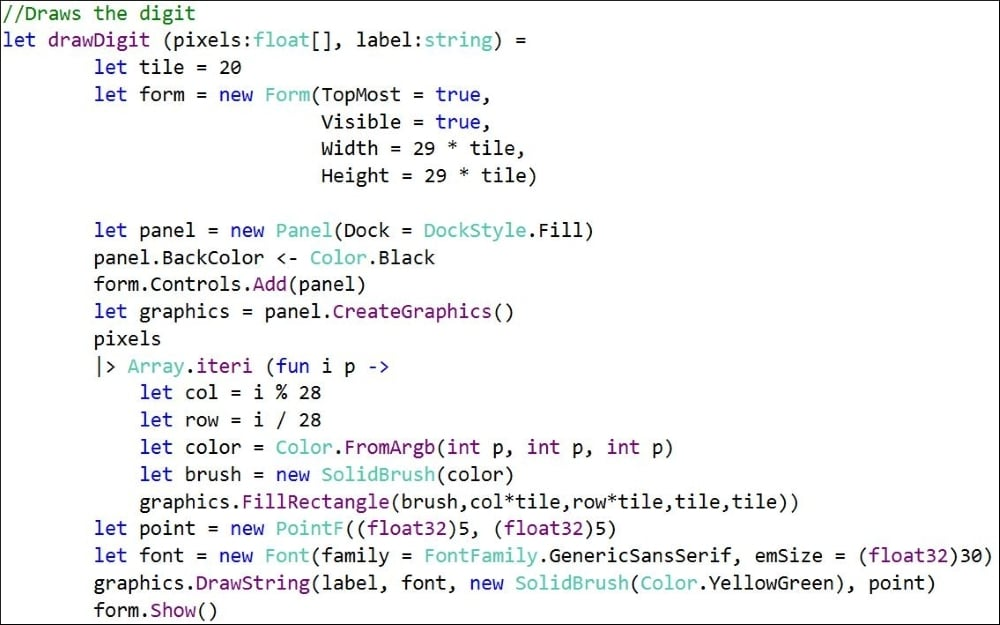6. Finally, in the `main` method, add the following code: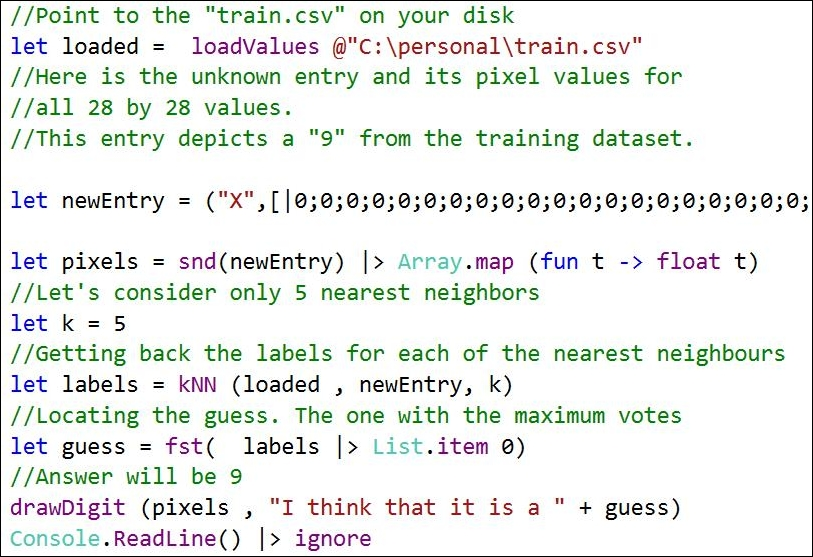When this program runs, it will produce the following output: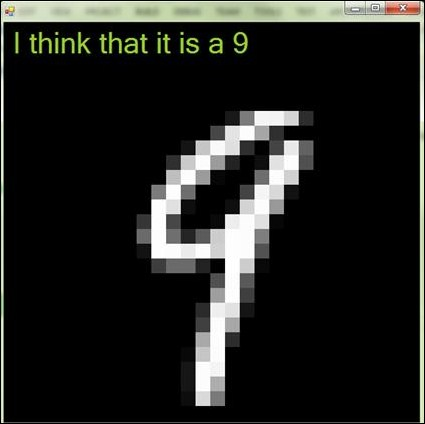### How does this work?

The `distance` function is based on the Euclidean distance function, as mentioned earlier in the chapter. Now you see that a general purpose Euclidean distance function is coded in the distance function. You might have noticed that there is a small difference between the formula and the implementation. The implementation finds the squared Euclidean distance given by the following formula: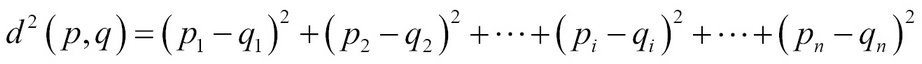Here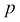and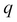denote the two vectors. In this case,might denote one example from the training set andmight denote the test example or the new uncategorized data that we have depicted by `newEntry` in the preceding code.

The `loadValues` function loads the pixel values and the category for each training/test data, and creates a list of `Entry` types from the CSV file.

The k-NN algorithm is implemented in the `kNN` function. Refer to the following line of code:

`|> List.map( fun x -> ( x.Label, distance  (x.Values, snd (newEntry) |>Array.toList )))`

This preceding code creates a list of tuples where the first element is the category of the entry and the second is the distance square value for the test data from each of the training entry. So it might look as follows: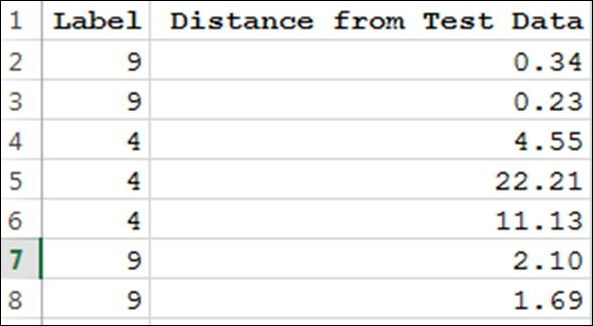Now consider the following line:

`|> List.sortBy ( fun x -> snd x)`

It sorts this list of tuples based on the increasing distance from the test data. Thus, the preceding list will become as shown in the following image: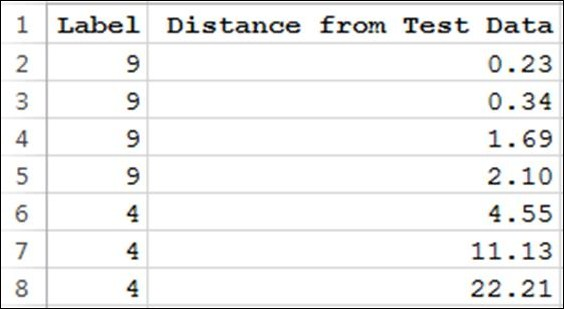If you see, there are four 9s and three 4s in this list. The following line transforms this list into a histogram:

`|> Seq.countBy (fun x -> fst x)`

So if k is chosen to be 5, then we will have four 9s and one 4. Thus, k nearest neighbor will conclude that the digit is probably a "9" since most of the nearest neighbors are "9".

The `drawDigit` function draws the digit pixel by pixel and writes out the guessed label for the digit. It does so by drawing each pixel on a tile size of 20.

## Summary

Sometimes one simple algorithm doesn't provide the needed accuracy. So then several methods are used and a special class of algorithm, known as Ensemble method, is used to club the individual results. In loose terms, it kind of resonates with the phrase "crowd-smart". You will learn about some ensemble methods in a later chapter.

I want to wrap up this chapter with the following tip. When you have a problem that you want to solve and you think machine learning can help, follow the following steps. Break the problem into smaller chunks and then try to locate a class of machine learning problem domain for this smaller problem. Then find the best method in that class to solve. Iterate over and over until your error rates are within permissible limits. And then wrap it in a nice application/user interface.

• ##### Sudipta Mukherjee

Sudipta Mukherjee was born in Kolkata and migrated to Bangalore. He is an electronics engineer by education and a computer engineer/scientist by profession and passion. He graduated in 2004 with a degree in electronics and communication engineering.

He has a keen interest in data structure, algorithms, text processing, natural language processing tools development, programming languages, and machine learning at large. His first book on Data Structure using C has been received quite well. Parts of the book can be read on Google Books. The book was also translated into simplified Chinese, available from Amazon.cn. This is Sudipta's second book with Packt Publishing. His first book, .NET 4.0 Generics , was also received very well. During the last few years, he has been hooked to the functional programming style. His book on functional programming, Thinking in LINQ, was released in 2014. He lives in Bangalore with his wife and son.

Sudipta can be reached via e-mail at [email protected] and via Twitter at @samthecoder.

Browse publications by this author

### Latest Reviews

(1 reviews total)